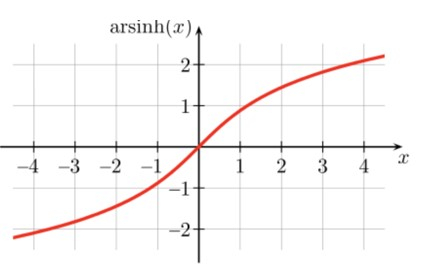# How to find the Hyperbolic ArcSine of a given value in Golang?

In this tutorial, we will learn how to find the Hyperbolic Arc Sine of a given value in the Golang programming Language. Golang language has many packages with predefined functions that the developer can use without writing the complete logic.

To perform the mathematical operations and logic we have a math package in Golang. We will use this package only to find the Hyperbolic Arc Sine of a given value. We will also see how to import the package and also how to call a function this package consists of by writing a Golang code.

## Hyperbolic Arc Sine

### Definition

Hyperbolic Arc Sine is a function similar to trigonometry functions. Hyperbolic Arc Sine is equal to the analogs of the trigonometric function. The formula for Hyperbolic Arc sine is written below and the value of Hyperbolic Arc Sine is at different angles.

### Syntax

asinh(x) = ln(x + √x^2 + 1)


### Graph## Value of Hyperbolic Arc Sine at different angles

• asinh(0) = 0

• asinh(30) = 4.094622224331

• asinh(45) = 4.499933104264

• asinh(60) = 4.787561179994

• asinh(90) = 5.192987713659

### Algorithm

Step 1 - Declaring the variable to store the value of the guardian and answer of float32 type.

Step 2 - Initializing the variable of a value.

Step 3- Call the function of Hyperbolic Arc sine and pass the value.

Step 4 - Printing the result.

### Example

In this example, we will write a Golang program in which we will import a math package and call the Hyperbolic Arc sine function.

package main
import (

// fmt package provides the function to print anything
"fmt"

// math package provides multiple functions for different

// mathematical operations
"math"
)
func main() {

// declaring the variables to store the value of \value and answer
fmt.Println("Program to find the Hyperbolic Arc sine of a given value in the Golang programming language using a math package.")

// initializing the value of the variable value
value = 4.5

// finding Hyperbolic Arc sine for the given value

// printing the result
fmt.Println("The Hyperbolic Arc sine value with the value of", value, "is", answer)
}


### Output

Program to find the Hyperbolic Arc sine of a given value in the Golang programming language using a math package.
The Hyperbolic Arc sine value with the value of 4.5 is 2.209347708615334


### Algorithm

Step 1 - Declaring the variable to store the value of the guardian and answer of float32 type.

Step 2 - Initializing the variable of a value.

Step 3 - Call the function of Hyperbolic Arc sine defined by us and pass the value as a parameter

Step 4 - Printing the result.

### Example

In this example, we will write a Golang program in which we will import a math package and call the Hyperbolic Arc sine function in a separate function and call that function main.

package main
import (

// fmt package provides the function to print anything
"fmt"

// math package provides multiple functions for different

// mathematical operations
"math"
)

// this is a function with a parameter of float64 type and a return type of float64
func HyperbolicArcSine(angle float64) float64 {

// returning the Hyperbolic Arc Sine of the angle
return math.Asinh(angle)
}
func main() {

// declaring the variables to store the value of value and answer
fmt.Println("Program to find the Hyperbolic Arc Sine of a given value in the Golang programming language using a separate function in the same program.")

// initializing the value of the value
value = 1

// finding Hyperbolic Arc sine for the given value in a separate function

// printing the result
fmt.Println("The Hyperbolic Arc Sine value with the value of", value, "is", answer)
}


### Output

Program to find the Hyperbolic Arc Sine of a given value in the Golang programming language using a separate function in the same program.
The Hyperbolic Arc Sine value with the value of 1 is 0.8813735870195432


## Conclusion

These are two ways to find the Hyperbolic Arc sine by using the function in the math package and passing the value as a parameter. The second approach will provide abstraction in the program. To learn more about Golang you can explore these tutorials.